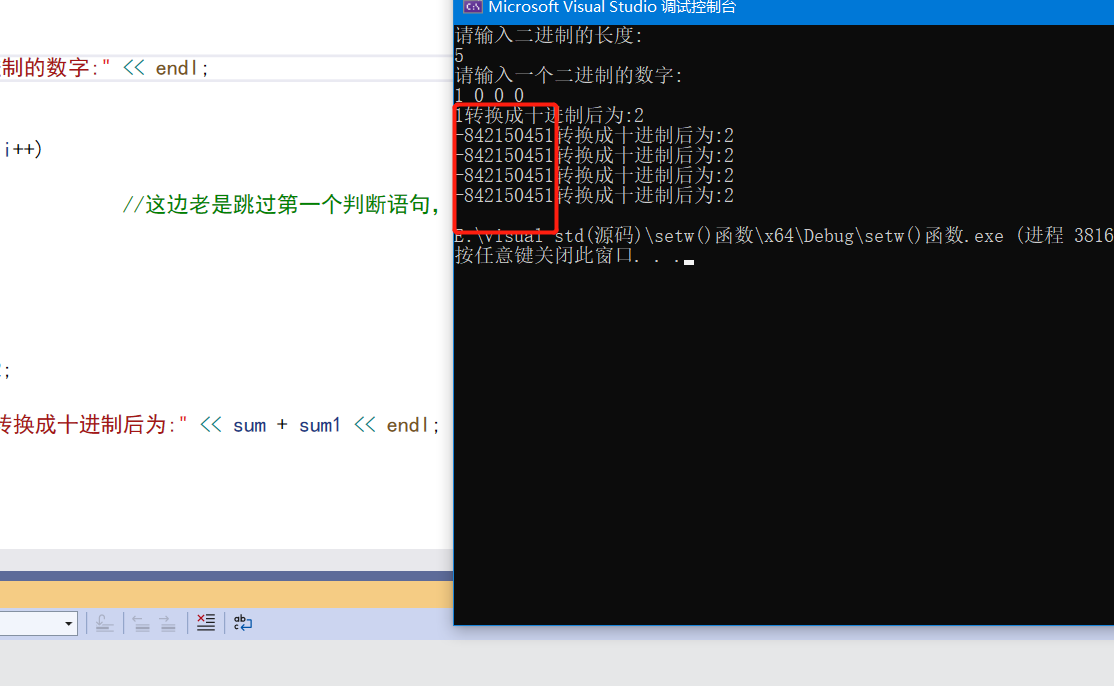浏览 26

# 【c++中for循环怎么调用动态数组?】调用数组中第二个元素时会出现乱码。

#include
using namespace std;
#include
int main()
{
int sum = 0, sum1 = 0, n;
int *p;
cout << "请输入二进制的长度:" << endl;
cin >> n;
p = new int[n];
cout << "请输入一个二进制的数字:" << endl;
cin >> *p;
int s1 = { 0 };
for (int i = 0; i < n; i++)
{
if (p[i] == 0) //这边老是跳过第一个判断语句，直接进行第二个语句
{
sum = sum + 1;
}
else
{
sum1 = sum1 + 2;
}
cout << *p << "转换成十进制后为:" << sum + sum1 << endl;
}
delete[]p;
return 0;
}• 写回答

#### 悬赏问题

• ¥15 关于#python#的问题，请各位专家解答！
• ¥100 关于远控软件的两个问题